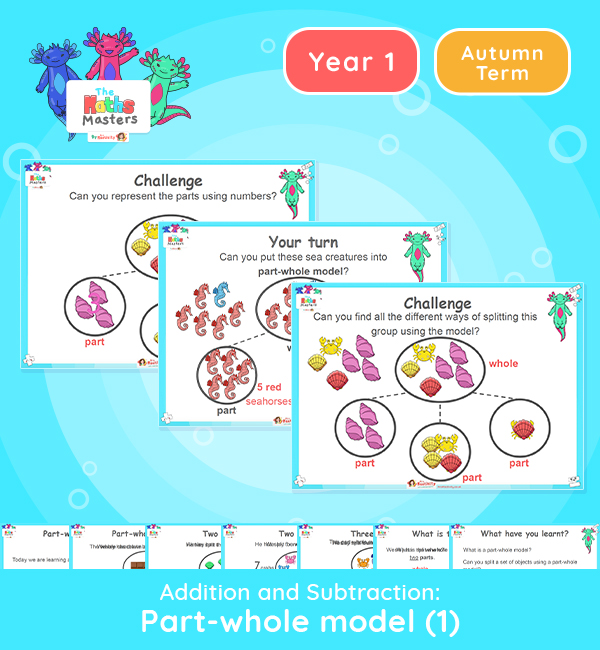# Year 1 | Part Whole Model 1 Lesson Presentation## Year 1 addition and subtraction resources

This lesson introduces the children to the part-whole model using images of objects.

Aligned with the maths mastery approach, this Year 1 | Part Whole Model 1 Lesson Presentation is fully editable, and is designed for the Year 1 maths curriculum to cover the following curriculum objectives:

Small Steps: Part-whole model with images

NC Links: Solve one step problems that involve addition and subtraction, using concrete objects and pictorial representations and missing number problems.

*Note this lesson is the prior learning to teaching about addition and subtraction, but the children will be adding and subtracting whilst forming parts and wholes.

Ready-to-progress criteria:  1AS-1 Compose numbers to 10 from 2 parts and partition numbers to 10 into parts

Aligned with the order of teaching of the maths mastery approach, use this to help your pupils get to grips with each mathematical concept. This lesson presentation also includes varied fluency activities, problem solving, and mathematical discussion questions too.

Explore our other year 1 addition and subtraction resources.

## Recently Viewed